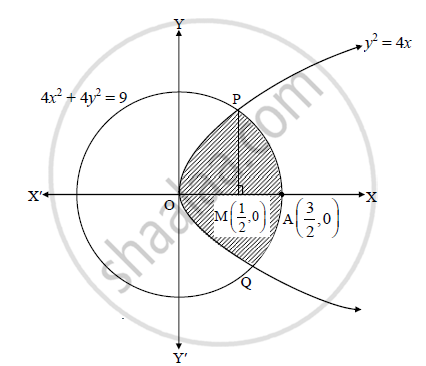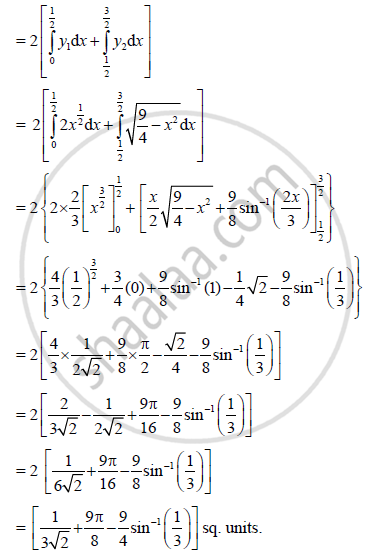HSC Arts 12th Board ExamMaharashtra State Board
Share

# Find the Area of the Region Bounded by the Curves Y2 = 4x and 4x2 + 4y2 = 9 with X >= 0. - HSC Arts 12th Board Exam - Mathematics and Statistics

ConceptPair of Straight Lines Point of Intersection of Two Lines

#### Question

Find the area of the region bounded by the curves y2 = 4x and 4x2 + 4y2 = 9 with x >= 0.

#### SolutionRequired area is nothing but area bounded by the parabola y2 = 4x and the circle x2 + y2 = 9/4

To find the points of intersection.

Solving the given equations, we get

x^2 + 4x - 9/4 = 0

∴ 4x2 + 16x − 9 = 0

∴ 4x2 + 18x − 2x − 9 = 0

∴ (2x − 1)(2x + 9) = 0

∴ x = 1/2 or x  = -9/2 (not possible)

When x = 1/2 , y = +- sqrt2

∴ The curves intersect at P(1/2, sqrt2) and Q(1/2, -sqrt2)

Consider, y2 = 4x

∴ y = 2x^(1/2) = y_1 ....(say)

Also, x^2 + y^2 = 9/2

:. y^2 = 9/4 - x^2

∴ y = sqrt(9/4 - x^2) = y_2 ...(say)

∴ Required area = A(OPAQO) =2.A(OPAMO)

= 2[A(OPMO) + A(PAMP)]Is there an error in this question or solution?

#### APPEARS IN

2012-2013 (October) (with solutions)
Question 5.2.3 | 4.00 marks

#### Video TutorialsVIEW ALL 

Solution Find the Area of the Region Bounded by the Curves Y2 = 4x and 4x2 + 4y2 = 9 with X >= 0. Concept: Pair of Straight Lines - Point of Intersection of Two Lines.
S